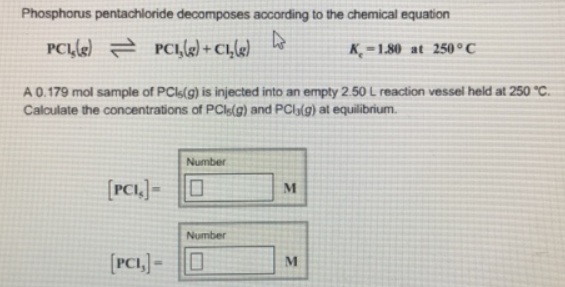# Problem: Phosphorus pentachloride decomposes according to the chemical equation PCl5 (g) ⇌ PCl3 (g) + Cl2 (g)    Kc = 1.80 at 250°C A 0.179 mol sample of PCI5(g) is injected into an empty 2.50 L reaction vessel held at 250°C. Calculate the concentrations of PCl5(g) and PCl3(g) at equilibrium.

###### FREE Expert Solution
86% (467 ratings)###### Problem Details

Phosphorus pentachloride decomposes according to the chemical equation

PCl5 (g) ⇌ PCl3 (g) + Cl2 (g)    Kc = 1.80 at 250°C

A 0.179 mol sample of PCI5(g) is injected into an empty 2.50 L reaction vessel held at 250°C. Calculate the concentrations of PCl5(g) and PCl3(g) at equilibrium.